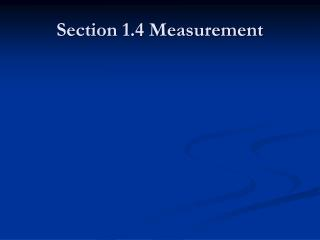DownloadDownload PresentationSection 1.4 Measurement

# Section 1.4 Measurement

Télécharger la présentation## Section 1.4 Measurement

- - - - - - - - - - - - - - - - - - - - - - - - - - - E N D - - - - - - - - - - - - - - - - - - - - - - - - - - -
##### Presentation Transcript

1. Section 1.4 Measurement

2. 12) How do you measure Energy? • 4.1 WORK, POWER, AND HORSEPOWER • Work is force applied over a distance. Work is expressed in units such as foot-pounds (ft.-lb.) or inchpounds For example, if a 10 pound weigh[ is lifted one foot, the work accomplished equals IO footpounds.

3. When a 10 pound weight is lifted one foot, 10 foot-pounds of work is done. Work = Force x Distance14 • Horsepower is used for stating mechanical power. Electrical machines such as motors are rated in horsepower. One horsepower (hp) is defined as a work rate of 550 ft.-lb./second. Also, 33,000 ft.-lb./min. equals one horsepower.

4. In Electricity, the unit of power is stated in Watts (W). It was named in honor of James Watt, who is credited with the invention of the steam engine. When 1 volt of electrical pressure moves 1 coulomb of electricity in 1 second, the work accomplished is equal to 1 Watt of power. Power = Work = Force x Distance Time Time

5. Recall the definition of the ampere: the movement of one coulomb of electrons past a given point in a circuit in one second. Thus, power in an electrical circuit can be written in terms of voltage and current. The formula for electrical power is: • P (watts) = E (volts) x I (amperes)

6. To convert electrical power, in watts, into mechanical power, in horsepower, you can use the following conversion factor. • 746 watts= 1 horsepower

7. The power formula, sometimes called Watt's law, can be arranged algebraically. If two quantities are known, the third unknown can be found. Use the device in Figure 4-2 to help memorize the following formulas. • P=I x E or I=P/E or E= P/I

8. Efficiency • It is the comparison of the power input and the power output. the closer you can get your output to match your input then you have better efficiency. What hurts efficiency?

9. Review Questions for Section 4.1 • 1. Define work. • 2. Define power. • 3. What is the symbol for mechanical power? • 4. What is the unit of electrical power? • 5. Define horsepower.

10. 6.State Watt's law. Use it in a formula with a given voltage and current. • 7. Compute the voltage and the power for the following figure. • 8. An electromechanical load is rated at 2238 watts. What is the equivalent rating in horsepower?

11. Practical Application • Observe Motors and Generators • gather the materials to do Motor vs Generator Activity

12. End